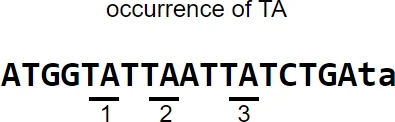# Python count() function for strings

In this article, you will learn how to use the in-built `count()` function for strings to count the number of non-overlapping occurrences of characters or substrings in a given input string.

Syntax: `count(substring, start, end)`

substring: characters or substring to search within a given string. It is required.
start : position to start a search within a string (default is 0). It is optional.
end : position to end search within a string (default is string end). It is optional.

It returns an integer value of count

`count()` function is case-sensitive, and hence the count of “A” or “a” is not the same

Let’s see some examples of `count()` function

### 1. Count the number of occurrences of characters

``````# create any random string
dna = "ATGGTATTAATTATCTGAta"
``````

In this string, count the number of occurrences of T character

``````dna.count("T")
# output
8
``````

### 2. Count the number of occurrences of substring

Count the number of occurrences of TA substring

``````dna.count("TA")
# output
3
``````### 3. Count the number of occurrences of substring using start and end positions

Count the number of occurrences of TA substring starting from 6th position to end position

``````# in Python, index starts at 0
dna.count("TA", 5)
# output
2
``````

Count the number of occurrences of TA substring starting from 6th position to 10th position

``````# in Python, index starts at 0
dna.count("TA", 5, 9)
# output
1
``````

### 4. `count()` function is case-sensitive

Count the number of occurrences of ta substring

``````dna.count("ta")
# output
1
``````

## Subscribe to get new article to your email when published

* indicates required